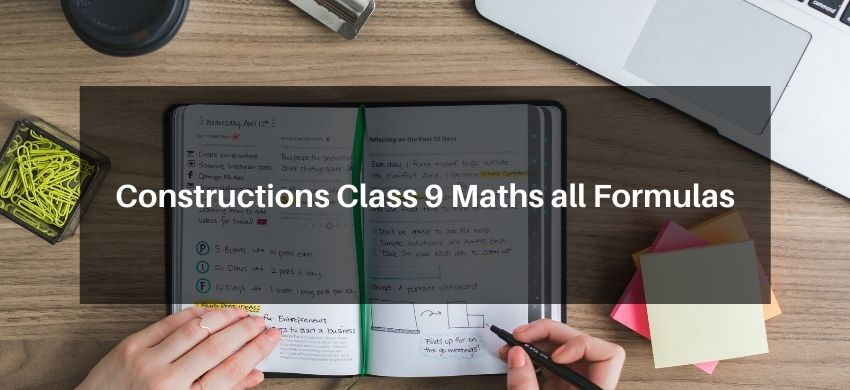# Constructions Class 9 Maths All FormulasHey, students are you looking for Constructions Class 9 Maths all Formulas? If yes. Then you are at the right place. In this post, I have listed all the formulas of Constructions Class 9 that you can use to learn and understand the concepts easily.

If you want to improve your class 9 Math, Constructions concepts, then it is super important for you to learn and understand all the formulas.

By using these formulas you will learn about the Constructions concepts for class 9.

With the help of these formulas, you can revise the entire chapter easily.

## Consturctions Class 9 Maths all Formulas

• A graduated scale, on one side of which centimeters and millimeters are marked off and on the other side inches are marked off.
• A pair of set-squares, one with angles $90^{\circ}, 60^{\circ}$ and $30^{\circ}$ and other with angles $90^{\circ}, 45^{\circ}$ and $45^{\circ}$
• A divider.
• A compass with provision of fitting a pencil at one end.
• A protractor.
But a geometrical construction is the process of drawing a geometrical figure using only two instruments-an un graduated ruler and a compass. In construction where measurements are also required, one may use a graduated scale and protractor also.

### Basic Constructions

• To construct the bisector of a given angle.
• To construct the perpendicular bisector of a given line segment.
• To construct and angle of $30^{\circ}, 45^{\circ}, 60^{\circ}, 90^{\circ}, 120^{\circ} \mathrm{m}$ (the initial point of a given ray.

### Construction of Triangles

• To construct a triangle given its base, a base angle and sym of the other two sides.
• To construct a triangle given its base, a base angle and the difference of the other two sides.
• To construct a triangle, given its perimeter and its two base angles.